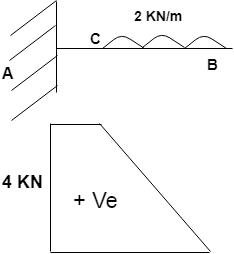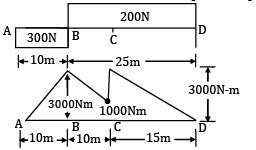Courses

# Test: SFD & BMD Level - 2

## 20 Questions MCQ Test Strength of Materials (SOM) | Test: SFD & BMD Level - 2

Description
This mock test of Test: SFD & BMD Level - 2 for Mechanical Engineering helps you for every Mechanical Engineering entrance exam. This contains 20 Multiple Choice Questions for Mechanical Engineering Test: SFD & BMD Level - 2 (mcq) to study with solutions a complete question bank. The solved questions answers in this Test: SFD & BMD Level - 2 quiz give you a good mix of easy questions and tough questions. Mechanical Engineering students definitely take this Test: SFD & BMD Level - 2 exercise for a better result in the exam. You can find other Test: SFD & BMD Level - 2 extra questions, long questions & short questions for Mechanical Engineering on EduRev as well by searching above.
QUESTION: 1

### Match List-I with List-II and select the correct answer using codes given below the lists.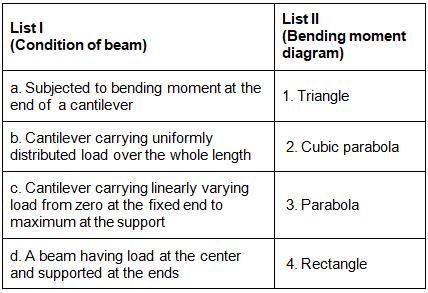Codes: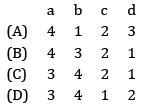Solution: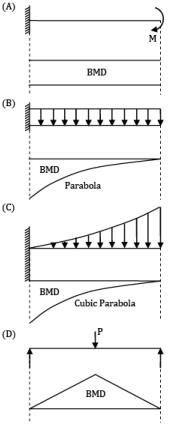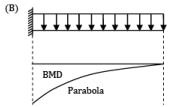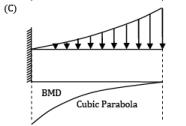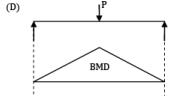QUESTION: 2

### A step in the bending moment diagram indicates then

Solution: A step in the bending moment diagram indicates, there is a couple acting at that section. A step in the shear force diagram indicates there is a concentrated load at that section.

QUESTION: 3

### If the shear force acting at every section of a beam is of the same magnitude and of the same direction then it represents a

Solution:
QUESTION: 4

If the bending moment at a section of a beam is zero then

Solution: At the point of zero bending moment shear force need not be maximum.

QUESTION: 5

The nature of combined stress in a beam depends on the nature of (where P(axial load) M(Bending moment))

Solution: Combined stress depends on axial force, ‘P’ and bending moment ‘M’.

QUESTION: 6

A simply supported beam of span L, carries uniformly distributed load of intensity ‘q’ over one half of the span. Shear force at mid-span section is

Solution: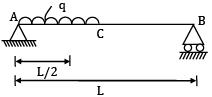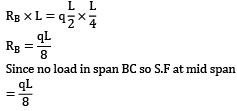Since no load in span BC so S.F at mid spanQUESTION: 7

A loaded beam is shown in the figure. The bending moment diagram of the beam is best represented as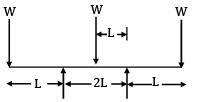Solution: Moment at Midpoint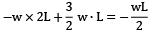But in options we are having positive moments at mid-point, so assume clockwise moment as positive and CCW moment as negative.

So, options could be (A), (C), (D).

In (C), (D) moment is becoming zero in between midpoint and endpoint which cannot happen. So, the answer is (A).

QUESTION: 8

The shearing force diagram for a beam is shown in the above figure. The bending moment diagram is represented by which one of the following?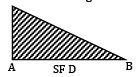Solution:
• As the SFD is linear, BMD should be parabolic.

• S. F = d(BM)/dx

• Slope at ‘A’ should be maximum as S.F is maximum.

• For ‘B’ the condition is satisfied.

QUESTION: 9

The figure shows the shear force diagram for an overhanging beam ACDB.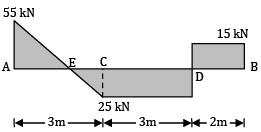Consider the following statements with respect to the above beam

1. The beam has supports at A and D

2. The beam carries a concentrated load at C of 25 kN

3. Bending moment at D is 15 kNm

4. The beam carries a uniformly distributed load of 80 kN over the portion AC.

Which of the statements given above is/are correct?

Solution: 1. Beam has support at A and D.

4. Beam carries a UDL of 80 kN over portion AC.

QUESTION: 10

A central concentrated load causes a maximum bending moment M in a simply supported beam. If the same load is distributed uniformly over the entire span, the maximum bending moment is

Solution: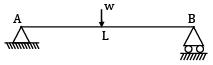M =WL / 4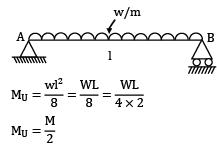QUESTION: 11

Point of inflection is the point where

Solution:
QUESTION: 12

Which of the following statement are true

I. At the point of concentrated load, there is a change in shear load in SFD and slope of curve is BMD

II. At the point of concentrated moment, there is no change in shear load in SFD but there is a change in moment in BMD

Solution:
QUESTION: 13

Beam A B C is 8 m long and is simply supported at A and at a point B such that AB = 6m. A point load of 1.5 T acts at C and a total u. d. l of 8 T acts over half of AB. The shear force at A in Tons would be

Solution: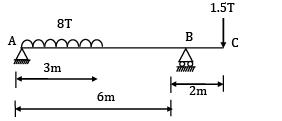Taking moment at B, ∑MB = 0

RA × 6 − 8 x 4.5 + 1.5 x 2 = 0

RA = 5.5T

QUESTION: 14

A beam with length ‘l’ between the pinned supports overhangs by ‘a’ and both sides. Then mid-span bending moment in the beam is zero under uniformly distributed load covering the entire span, if

Solution:RA + RB = w x (l + 2a)

RA = RB = 1 /2∙ w(l + 2a)

Mmid = 0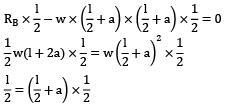l = 2a

QUESTION: 15

The loading on a simply supported beam of span 10 m is given as u. d. l, q = 20 kN/m, 4m ≤ x ≤ 10 m. The shear force at mid-span section is

Solution: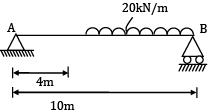RA × 10 = 20 x 6 x 6/2

RA = 36kN

RB = 120 − 36 = 84kN

S.F at mid span = −36 + 20

= −16kN

Or

= 84 − 100 = −16kN

QUESTION: 16

A wooden log of length 5m is floating in water. Its self-weight is 1kN/m. If a man of weight 500 N is standing at the mid span, the maximum B.M. is

Solution: Assuming that Buoyancy force exerted by water is UDL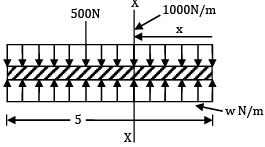ERy = 0 ⇒ 500 + 1000 x 5 = w x 5

w = 1100 N/m

Moment equation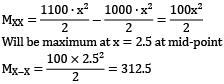QUESTION: 17

The given figure shown a beam BC simply supported at C and hinged at B (free end) of a cantilever AB. The beam and the cantilever carry forces of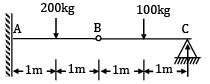100kg and 200kg are respectively. The bending moment at B is

Solution: The BM at the internal hinge will always be zero.

QUESTION: 18

A beam subjected to a load P is shown in the given figure. The bending moment at the support AA of the beam will be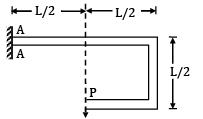Solution: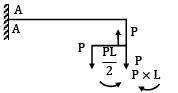Load P at end produces moment PL/2 in anticlockwise direction. Load P at the end produces a moment of PL in clockwise direction. Net moment at AA is PL/2.

QUESTION: 19

How do point loads and udl be represented in SFD?

Solution:

According to BIS, the standard symbols used for sketching SFD are